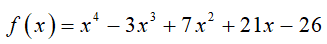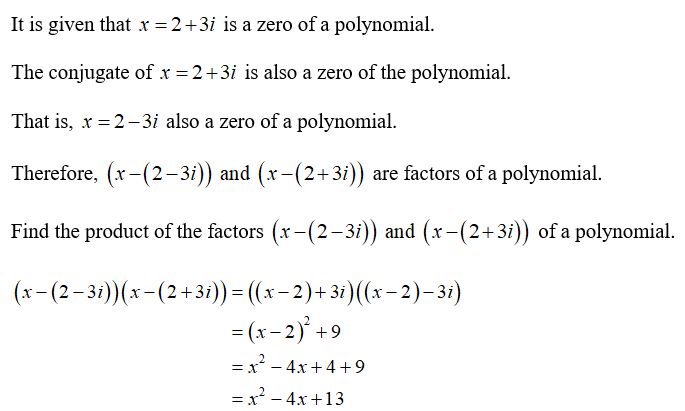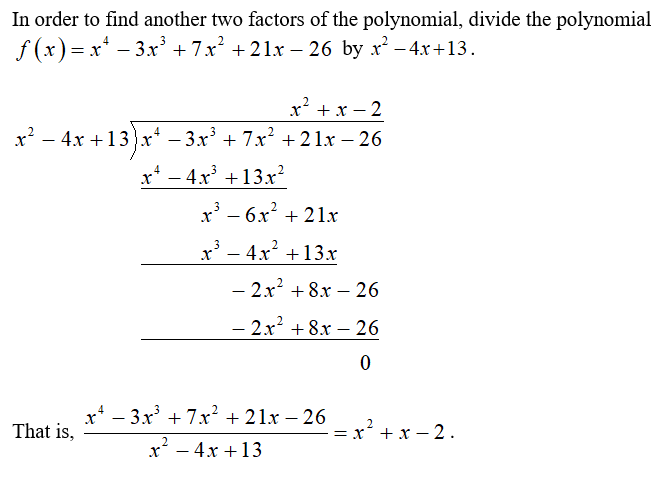# Find all complex zeros of the following polynomial given that 2+3i is a zero.f(x)=x4-3x3+7x2+21x-26

Question
4 views

Find all complex zeros of the following polynomial given that 2+3i is a zero.

f(x)=x4-3x3+7x2+21x-26

check_circle

The polynomial is given bySince the highest degree of a polynomial is 4, it have 4 roots.Obtain the other factors of a polynomial....

### Want to see the full answer?

See Solution

#### Want to see this answer and more?

Solutions are written by subject experts who are available 24/7. Questions are typically answered within 1 hour.*

See Solution
*Response times may vary by subject and question.
Tagged in

### Calculus